• ## Kmeans聚类算法详解

万次阅读 多人点赞 2018-05-16 18:41:40
摘要：本文通过图文详细介绍Kmeans聚类算法的原理和程序实现，以及如何选取类簇中心点。本文首先介绍利用该算法的原理及理解，详细介绍基于MATLAB设计一个自定义的Kmeans函数过程，然后利用该函数对UCI的数据集进行...
摘要：本文详细介绍Kmeans聚类算法的原理和程序实现。首先介绍利用该算法的原理及理解，详细介绍基于MATLAB设计一个自定义的Kmeans函数过程，然后利用该函数对UCI的数据集进行聚类以测试聚类结果。后续章节将介绍的主要部分有：

Kmeans算法的原理及理解
编程实现
聚类结果评价
类簇中心点的选取

点击下载：本文Kmeans算法M函数及测试完整文件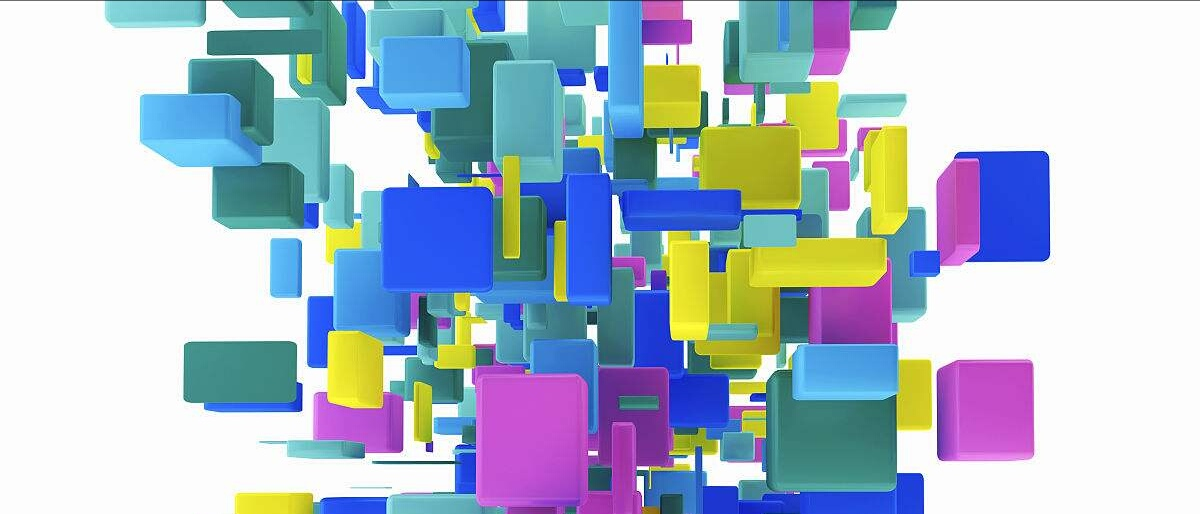1. 前言

作为无监督聚类算法中的代表——K均值聚类（Kmeans）算法，该算法的主要作用是将相似的样本自动归到一个类别中。所谓的监督算法，就是输入样本没有对应的输出或标签。聚类（clustering）试图将数据集中的样本划分为若干个通常是不相交的子集，每个子集称为一个“簇（cluster）”，聚类既能作为一个单独过程，用于找寻数据内在的分布结构，也可作为分类等其他学习任务的前去过程。——《Machine Learning》

聚类算法也许是机器学习中“新算法”出现最多、最快的领域，一个重要的原因是聚类不存在客观标准，给定数据集总能从某个角度找到以往算法未覆盖的某种标准从而设计出新算法。Kmeans算法十分简单易懂而且非常有效，但是合理的确定K值和K个初始类簇中心点对于聚类效果的好坏有很大的影响。众多的论文基于此都提出了各自行之有效的解决方案，新的改进算法仍然不断被提出，此类文章大家可以在Web Of Science中搜索。
尽管Kmeans算法在MATLAB、Python等语言的工具箱函数中都有自带的函数可供调用，但作为机器学习的研究者新来说要设计出新的算法，有时就得“定制”自己的Kmeans函数了。自己动手编写无疑也更加能理解算法的具体过程，接下来就让我们进入正题吧

2. Kmeans算法的原理与理解
Kmeans算法是最常用的聚类算法，主要思想是:在给定K值和K个初始类簇中心点的情况下，把每个点(亦即数据记录)分到离其最近的类簇中心点所代表的类簇中，所有点分配完毕之后，根据一个类簇内的所有点重新计算该类簇的中心点(取平均值)，然后再迭代的进行分配点和更新类簇中心点的步骤，直至类簇中心点的变化很小，或者达到指定的迭代次数。
2.1 基本原理
假定给定数据样本X，包含了n个对象$X=\left \{ X_{1} ,X_{2},X_{3},...,X_{n}\right \}$,其中每个对象都具有m个维度的属性。Kmeans算法的目标是将n个对象依据对象间的相似性聚集到指定的k个类簇中，每个对象属于且仅属于一个其到类簇中心距离最小的类簇中。对于Kmeans，首先需要初始化k个聚类中心$\left \{ C_{1} ,C_{2},C_{3},...,C_{k}\right \},1,然后通过计算每一个对象到每一个聚类中心的欧式距离，如下式所示$dis(X_{i},C_{j})=\sqrt{\sum_{t=1}^{m}(X_{it}-C_{jt})^{2}}$
上式中，$X_{i}$表示第i个对象$1\leq i\leq n$,$C_{j}$表示第j个聚类中心的$1\leq j\leq k$，$X_{it}$表示第i个对象的第t个属性，$1\leq t\leq m$，$C_{jt}$表示第j个聚类中心的第t个属性。
依次比较每一个对象到每一个聚类中心的距离，将对象分配到距离最近的聚类中心的类簇中，得到k个类簇$\left \{ S_{1},S_{2},S_{3},...,S_{k} \right \}$

Kmeans算法用中心定义了类簇的原型，类簇中心就是类簇内所有对象在各个维度的均值，其计算公式如下$C_{t}=\frac{\sum_{X_{i}\in S_{l}}X_{i}}{\left | S_{l} \right |}$
式中，$C_{l}$表示第l个聚类的中心，$1\leq l\leq k$，$\left | S_{l} \right |$表示第l个类簇中对象的个数，$X_{i}$表示第l个类簇中第i个对象，$1\leq i\leq\left | S_{l} \right |$。
2.2 算法流程

输入：样本集$D=\left \{ x_{1},x_{2},x_{3},...,x_{m} \right \}$；聚类簇数k.
过程：
1：从D中随机选择k个样本作为初始均值向量$\left \{ \mu _{1},\mu _{2},\mu _{3},...,\mu _{k} \right \}$
2：repeat
3：      令$C_{i}=\varnothing (1\leqslant i\leqslant k)$
4：     for j=1,2,…,m  do
5：           计算样本$x_{j}$与各均值向量$\mu_{i}(1\leqslant i\leqslant k)$的距离：$d_{ji}=\left \| x_{j}-\mu_{i} \right \|_{2}$;
6：            根据距离最近的均值向量确定$x_{j}$的簇标记：$\lambda _{j}=arg min_{i\in \left \{ 1,2,3,...,k \right \}}d_{ji}$；
7：            将样本$x_{j}$划入相应的簇：$C_{\lambda_{j}}=C_{\lambda_{j}}\cup \left \{ x_{j} \right \}；$
8：        end for
9：        for i=1,2,…,k do
10:               计算新均值向量：$\mu_{i}^{'}=\frac{1}{\left | C_{i} \right |}\sum _{x\in C_{i}}x$;
11：             if  $\mu_{i}^{'}\neq \mu_{i}$  then
12：                 将当前均值向量 $\mu_{i}$更新为$\mu_{i}^{'}$
13：             else
14：                 保持当前均值不变
15：                 end if
16：                 end for
17：until  当前均值向量均未更新
输出：簇划分$C=\left \{ C_{1} ,C_{2},...,C_{k} \right \}$

以上算法流程引自周志华《机器学习》，从流程来看K-means算法计算步骤基本上可以概括为两个部分：（1）计算每一个对象到类簇中心的距离；（2）根据类簇内的对象计算新的簇类中心。

3. 编程实现
为了方便应用我们将其编写为一个M函数KMeans()，首先需要确定函数的输入输出。这里输入参数为：data，K，iniCentriods，iterations（其中data为输入的不带标号的数据集数据矩阵，大小为numOfDatanumOfAttributes，K为数据分的类簇数目，iniCentriods为自行指定的初始聚类中心矩阵，大小为KnumOfAttributes，iterations为算法迭代次数。）
输出参数为：Idx，centroids，Distance（Idx为返回的分类标号， centroids为每一类的中心，Distance为类内总距离）
根据前面2.2节中的算法流程编写Kmeans算法的MATLAB程序如下
%% Kmeans算法
% 输入：
% data 输入的不带分类标号的数据
% K 数据一共分多少类
% iniCentriods 自行指定初始聚类中心
% iterations 迭代次数

% 输出：
% Idx 返回的分类标号
% centroids 每一类的中心
% Distance 类内总距离

function [Idx,centroids,Distance]=KMeans(data,K,iniCentriods,iterations)
[numOfData,numOfAttr]=size(data); % numOfData是数据个数，numOfAttr是数据维数
centroids=iniCentriods;
%% 迭代
for iter=1:iterations
pre_centroids=centroids;% 上一次求得的中心位置

tags=zeros(numOfData,K);
%% 寻找最近中心，更新中心
for i=1:numOfData
D=zeros(1,K);% 每个数据点与每个聚类中心的标准差
Dist=D;

% 计算每个点到每个中心点的标准差
for j=1:K
Dist(j)=norm(data(i,:)-centroids(j,:),2);
end

[minDistance,index]=min(Dist);% 寻找距离最小的类别索引
tags(i,index)=1;% 标记最小距离所处的位置（类别）
end

%% 取均值更新聚类中心点
for i=1:K
if sum(tags(:,i))~=0
% 未出现空类，计算均值作为下一聚类中心
for j=1:numOfAttr
centroids(i,j)=sum(tags(:,i).*data(:,j))/sum(tags(:,i));
end
else % 如果出现空类，从数据集中随机选中一个点作为中心
randidx = randperm(size(data, 1));
centroids(i,:) = data(randidx(1),:);
tags(randidx,:)=0;
tags(randidx,i)=1;
end
end

if sum(norm(pre_centroids-centroids,2))<0.001  % 不断迭代直到位置不再变化
break;
end

end

%% 计算输出结果
Distance=zeros(numOfData,1);
Idx=zeros(numOfData,1);
for i=1:numOfData
D=zeros(1,K);% 每个数据点与每个聚类中心的标准差
Dist=D;
% 计算每个点到每个中心点的标准差
for j=1:K
Dist(j)=norm(data(i,:)-centroids(j,:),2);
end

[distance,idx]=min(Dist);% 寻找距离最小的类别索引
distance=Dist(idx);

Distance(i)=distance;
Idx(i)=idx;
end
Distance=sum(Distance,1);% 计算类内总距离
end

在以上代码中其最主要部分在于第18至58行，进行寻找最近中心和求取均值更新聚类中心点。值得注意的是，在聚类过程中可能会出现空类即代码第44行那样，为保证算法的继续运行，从数据集中随机选取一个点作为中心。
4. 聚类结果评价
为了验证编写的Kmeans函数的性能，这里对想用的UCI数据集Iris数据集进行聚类并计算聚类的准确率，Iris数据集可以在http://archive.ics.uci.edu/ml/index.php上下载得到。首先读取Iris数据集，自行指定初始聚类中心调用前面编写的KMeans函数进行聚类，然后计算聚类的准确率，其代码如下
clear
data=load('Iris.txt');
data=data(:,2:end);

matrix=[5.9016,2.7484,4.3935,1.4339;6.8500,3.0737,5.7421,2.0711;5.0060,3.4280,1.4620,0.2460];
[Idx,C,distance]=KMeans(data,3,matrix,500);
Distance=sum(distance)

c1=Idx(1:50,1);c2=Idx(51:100,1);c3=Idx(101:150,1);
accuracy=(sum(c1==mode(Idx(1:50,1)))+sum(c2==mode(Idx(51:100,1)))+sum(c3==mode(Idx(101:150,1))))/150

为方便使用Iris数据集经过了一些整理，这里将最后一列的带字符串的标签Iris-setosa，Iris-versicolor，Iris-virginica分别用数字1,2,3代替并移到了第一列，所以第三行选取的是从第二列至最后一列的数据。第5行中的matrix是查阅论文得到的一个初始聚类中心，正好用来比对聚类结果。第6行则调用KMeans()函数进行聚类，得到聚类标号和类内距离。对每类的类内距离求和即得到总的距离Distance，如第7行。准确率的计算有点麻烦，因为不能直接用KMeans计算后得到的标号跟原数据集中的标号对比计算准确率，KMeans只需要也只能将那些“相似”的数据点聚集到一类中，而给这一类数据的标号却是可能跟原数据集不同的。
这里采用一个简单的方法，从原数据集的标签可以看出第1-50个数据点为一类（Iris-setosa），第51-100为一类（Iris-versicolor），第101-150为一类（Iris-virginica），因此只需确定每50个数据点中的聚类标号是不是一致。取它们之中数目最多的标号作为正确的个数，最终比上数据集的总数即为准确率。以上代码运行结果如下所示。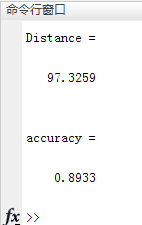5. 类簇中心点的选取
KMeans算法本身思想比较简单，但是合理的确定K值和K个初始类簇中心点对于聚类效果的好坏有很大的影响。最简单的确定初始类簇中心点的方法是随机产生数据大小范围内的K个点作为初始的簇类中心点。随机产生初始点并进行测试的程序代码如下
clear
data=load('Iris.txt');
data=data(:,2:end);
K=3;

%% 产生随机初始点
[numOfData,numOfAttr]=size(data);   % numOfData是数据个数，numOfAttr是数据维数

centroids=zeros(K,numOfAttr);       % 随机初始化，最终迭代到每一类的中心位置
maxAttr=zeros(numOfAttr);        % 每一维最大的数
minAttr=zeros(numOfAttr);        % 每一维最小的数
for i=1:numOfAttr
maxAttr(i)=max(data(:,i));    % 每一维最大的数
minAttr(i)=min(data(:,i));    % 每一维最小的数
for j=1:K
centroids(j,i)=maxAttr(i)+(minAttr(i)-maxAttr(i))*rand();  % 随机初始化，选取每一维[min max]中初始化
end
end

[Idx,C,distance]=KMeans(data,K,centroids,500);% 调用KMeans
Distance=sum(distance)% 计算类内距离之和

%% 计算准确率
c1=Idx(1:50,1);c2=Idx(51:100,1);c3=Idx(101:150,1);
Accuracy=(sum(c1==mode(Idx(1:50,1)))+sum(c2==mode(Idx(51:100,1)))+sum(c3==mode(Idx(101:150,1))))/numOfData

可以多运行几次以上代码，可以看出由于初始点事随机选取的每次运行得到的结果有所差异。这也是基本Kmeans算法的一个缺点，随着众多改进算法的提出Kmeans算法的这一问题也得到改善，深入了解的朋友可以查阅相关论文。
6. 结束语
本博文的完整MATLAB程序文件与整理好的数据集文件已经上传，下载即可运行。下载地址如下
点击下载：本文Kmeans算法M函数及测试完整文件
【公众号获取】
本人微信公众号已创建，扫描以下二维码并关注公众号“AI技术研究与分享”，后台回复“KM20180516”即可获取全部资源文件信息。由于编者能力有限，代码即使经过了多次校对，也难免会有疏漏之处。希望您能热心指出其中的错误，以便下次修改时能以一个更完美更严谨的样子，呈现在大家面前。同时如果有更好的实现方法也请您不吝赐教。


展开全文人工智能
• 多维kmeans聚类，有数据例程，有轮廓系数评价聚类效果
• Kmeans聚类可以支持2D和3D数据的处理，可以清晰观测聚类中心的移动过程，可以自选K的大小。
• KMeans聚类 1､工作原理 随机生成聚类中心，根据样本到聚类中心的距离进行分类，然后以各分类的样本中心为新的聚类中心，通过不断迭代更新聚类中心，以达到分类效果。 注： 1､KMeans聚类是根据聚类中心进行...
KMeans聚类
1､工作原理
随机生成聚类中心，根据样本到聚类中心的距离进行分类，然后以各分类的样本中心为新的聚类中心，通过不断迭代更新聚类中心，以达到分类效果。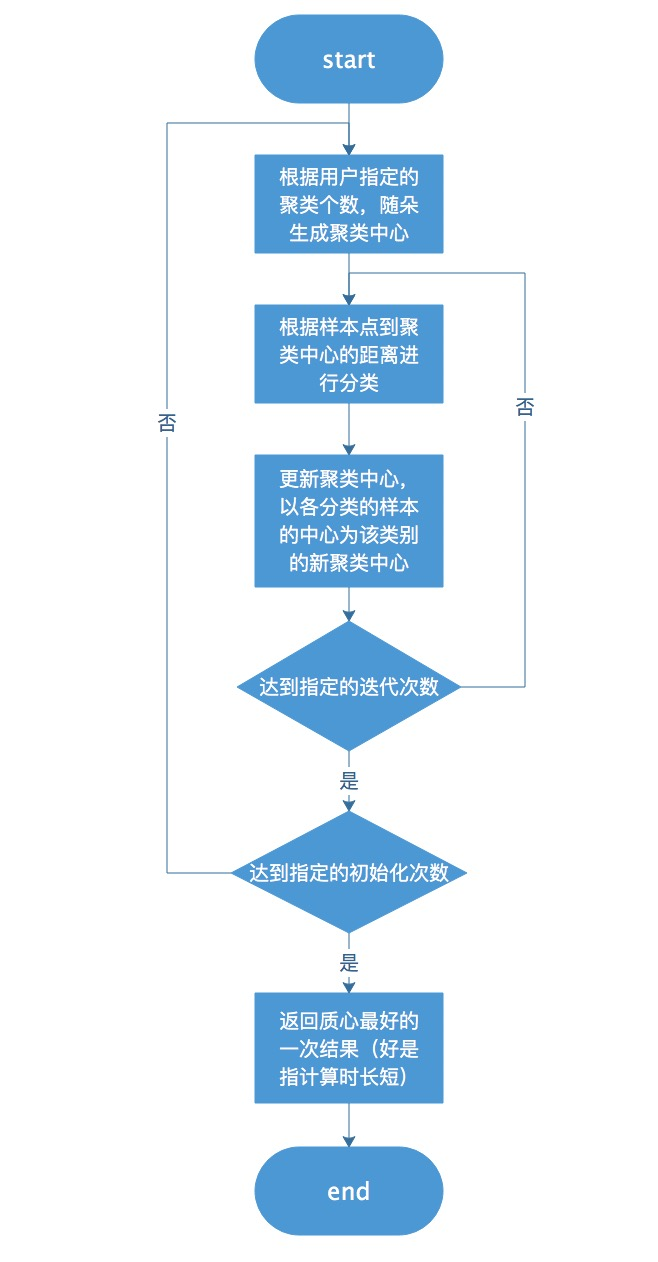注：
1､KMeans聚类是根据聚类中心进行分类的，所以需要指定聚类中心的个数，也就是分类的个数。
2､KMeans聚类质量的好坏依赖于初始的聚类中心点，可以通过多次初始化聚类中心，以达到选择好的聚类结果。
3､KMeans聚类迭代到最优聚类中心时，聚类中心可能会续断更新也可能不再更新，所以需要通过指定迭代次数来结束更新。
2､sklearn中的KMeans

##导入KMeans库
from sklearn.cluster import KMeans
##创建模型
kmeans = KMeans(n_clusters=2, random_state=0)
##拟合数据
kmeans.fit(X)
##预测
kmeans.predict([[0, 0], [4, 4]])
##获取聚类中心
kmeans.cluster_centers_

参数：
n_clusters：聚类中心的个数，默认值8；
n_init：初始化聚类中心的次数，默认值10；
max_iter：迭代次数，默认值300；
3､KMeans的优缺点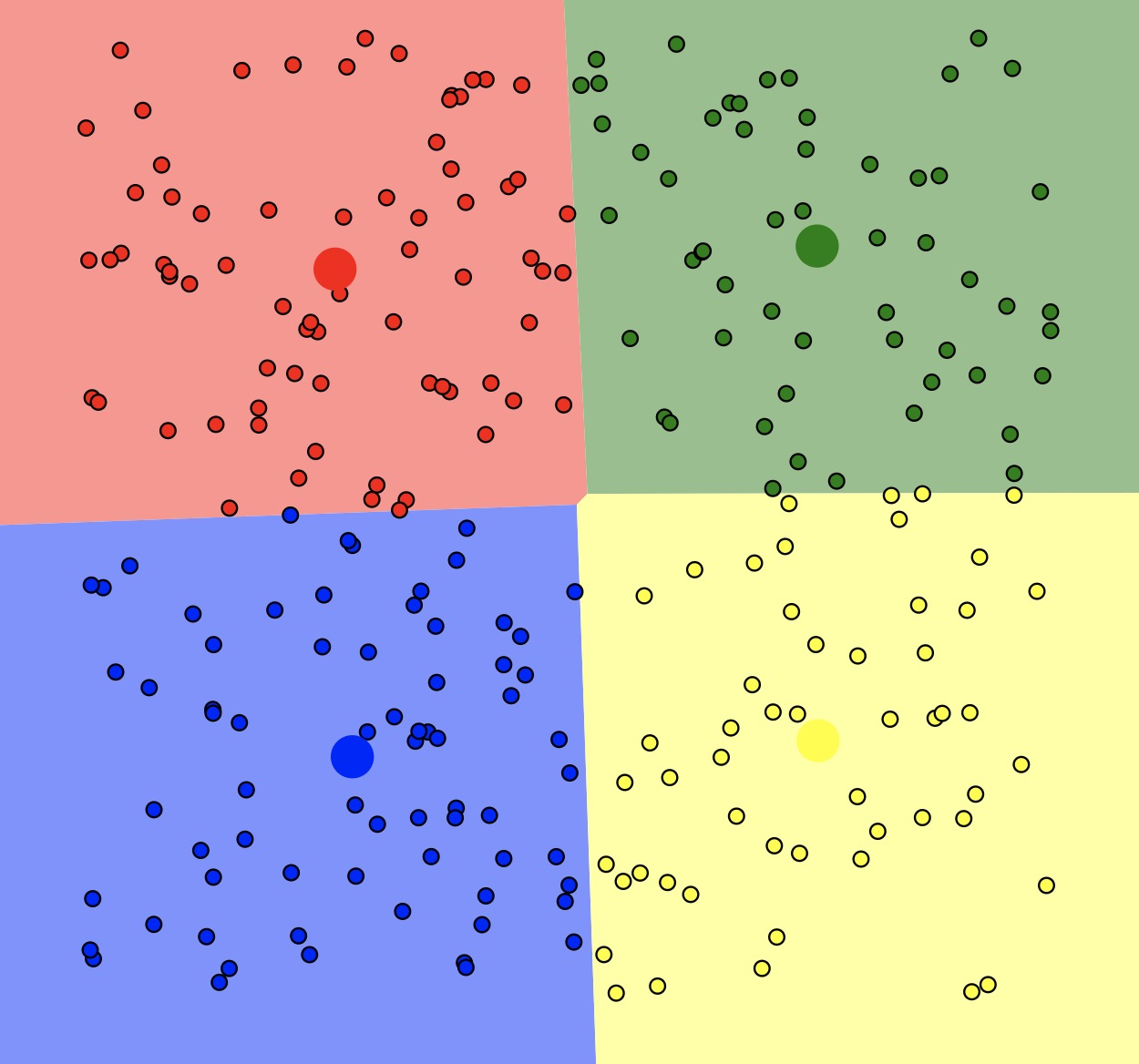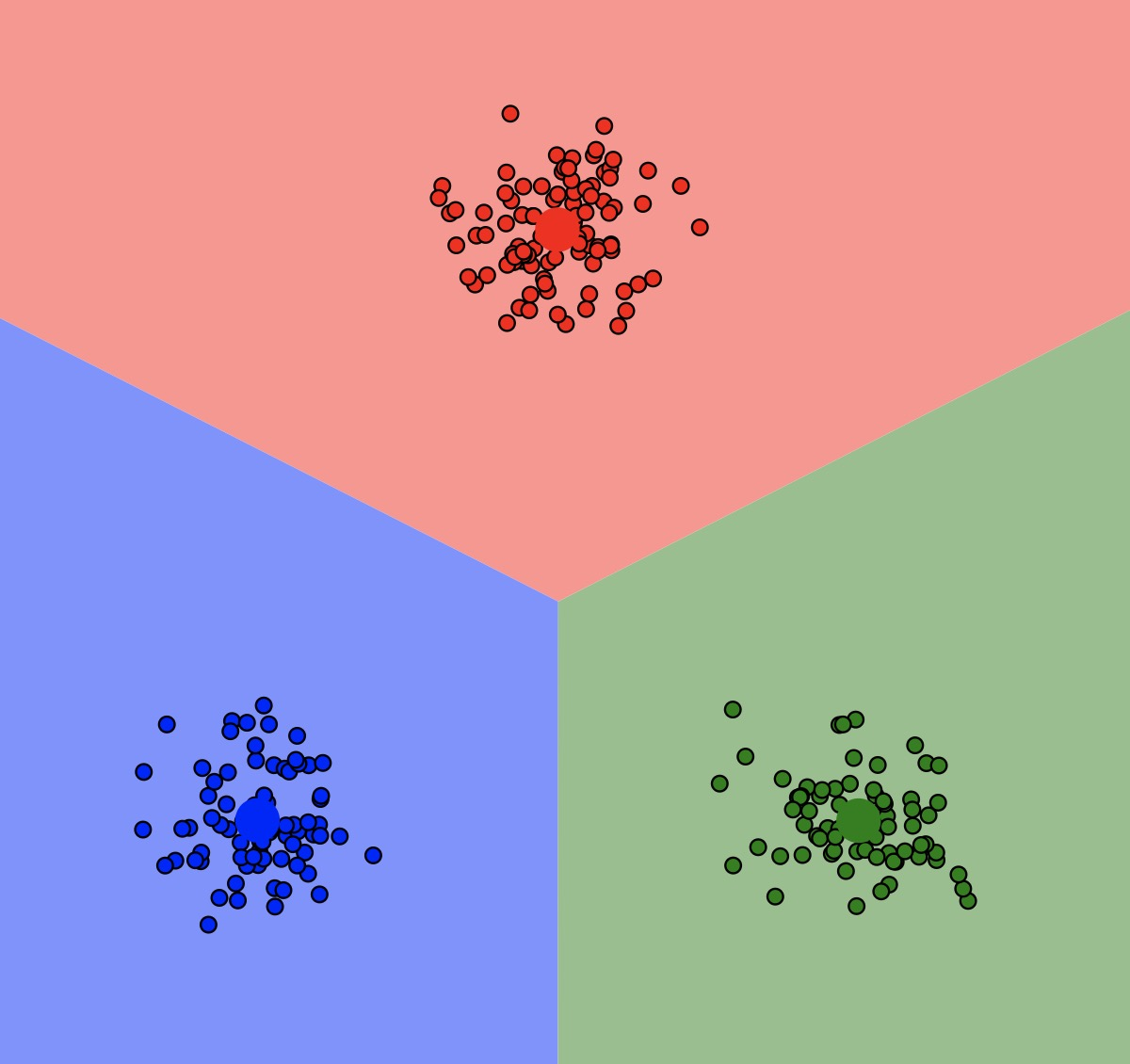$(图一）（图二)$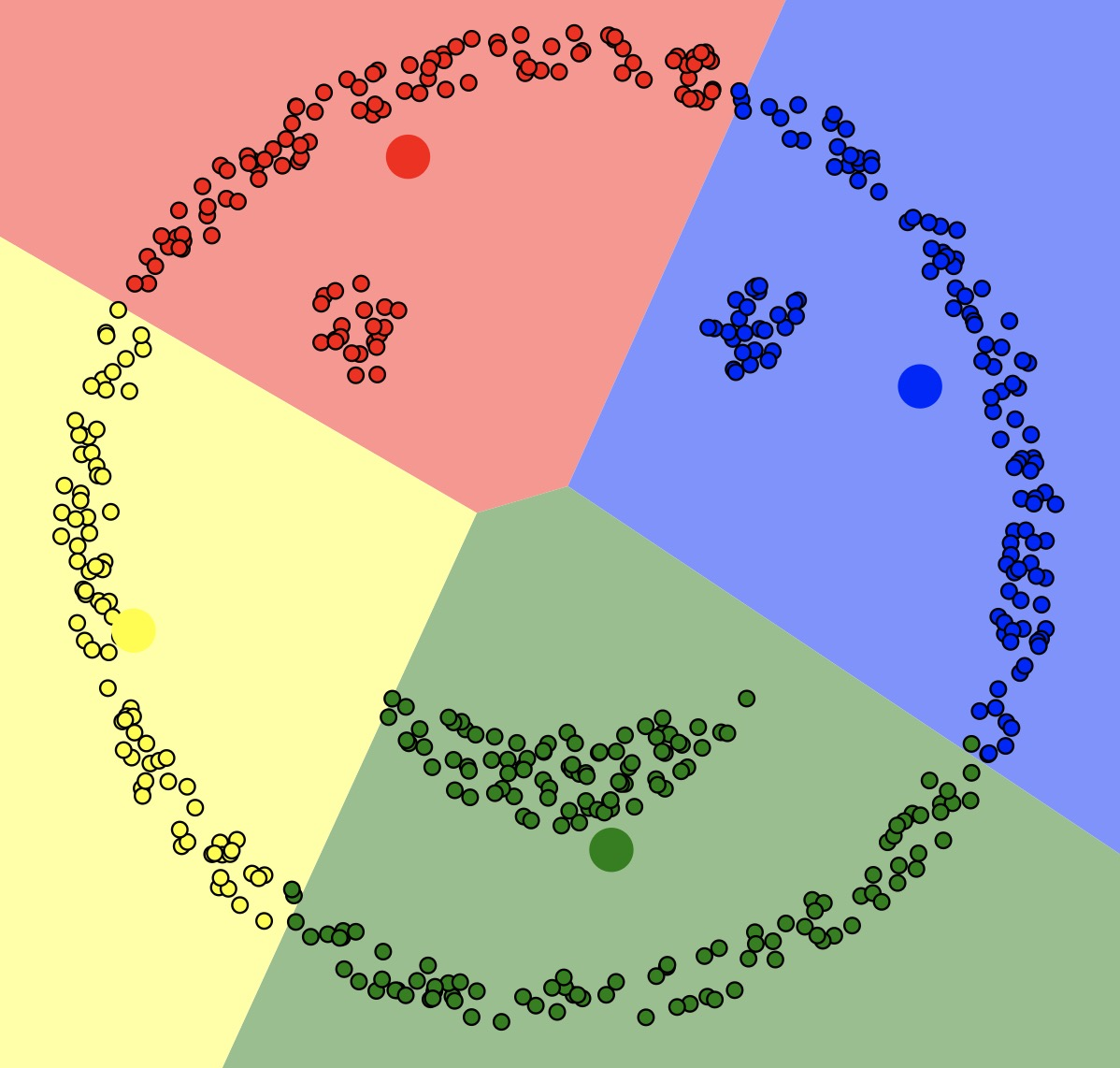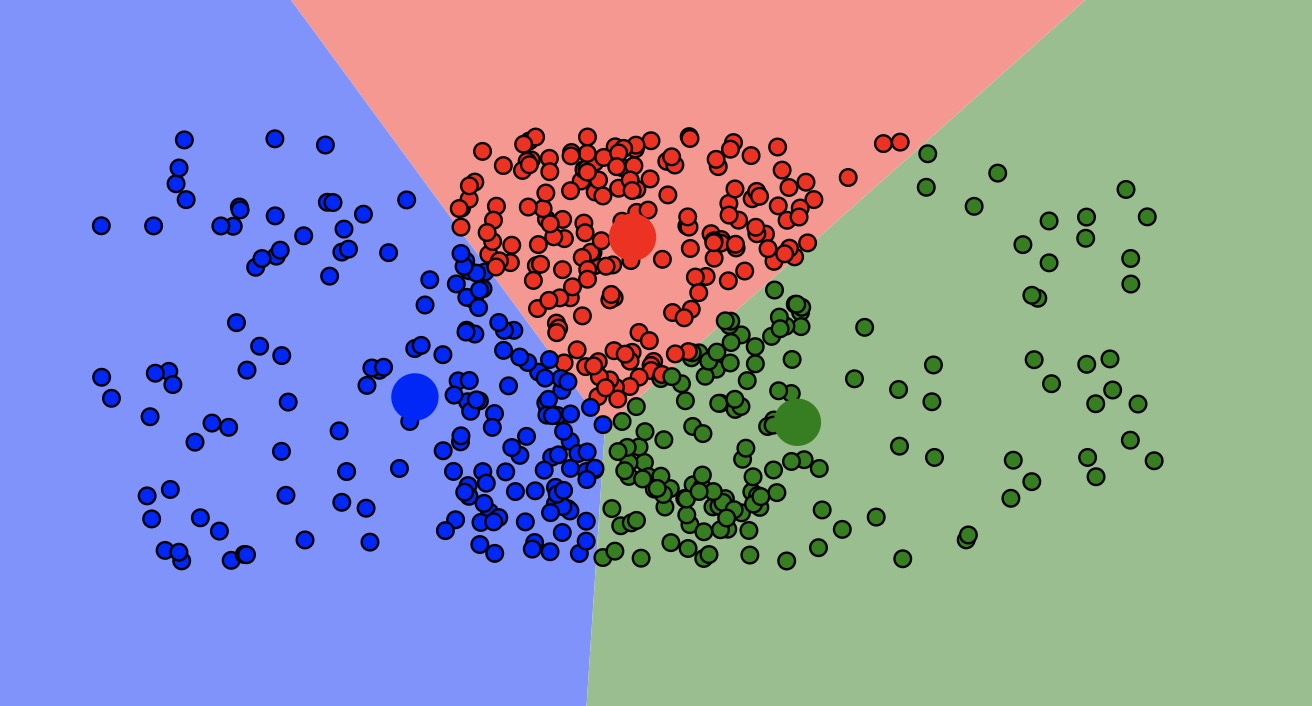$(图三）（图四)$
如上图所示，KMeans对于图一图二的聚类效果很好，但对于图三的聚类效果就很差了，图四的聚类效果非常依懒初始聚类中心的位置。


展开全文• kmeans聚类：一维数据的kmeans聚类算法的实现Python
• 基于KMeans聚类的协同过滤推荐算法可运用于基于用户和基于项目的协同过滤推荐算法中，作为降低数据稀疏度和提高推荐准确率的方法之一，一个协同过滤推荐过程可实现多次KMeans聚类。 一、基于KMeans聚类的协同过滤...
• 采用两个向量并执行 kmeans 聚类的简单通用函数。 有非常基本的示例代码来调用 kmeans 聚类算法和显示图。 基于来自 mathworks 网站和 matlab 文档的代码。matlab
• Kmeans聚类定义、KMeans聚类的步骤、Kmeans聚类常见问题及改进、Kmeans聚类的变形、Kmeans聚类的优缺点 目录 Kmeans聚类定义、KMeans聚类的步骤、Kmeans聚类常见问题及改进、Kmeans聚类的变形、Kmeans聚类的优...
Kmeans聚类定义、KMeans聚类的步骤、Kmeans聚类常见问题及改进、Kmeans聚类的变形、Kmeans聚类的优缺点

目录

Kmeans聚类定义、KMeans聚类的步骤、Kmeans聚类常见问题及改进、Kmeans聚类的变形、Kmeans聚类的优缺点

Kmeans聚类定义

KMeans聚类的步骤

Kmeans聚类常见问题及改进

Kmeans聚类的变形

Kmeans聚类的优缺点

Kmeans聚类定义

Kmeans聚类算法是划分聚类方法中最常用、最流行的经典算法，许多其他的方法都是k—means聚类算法的变种。Kmeans聚类算法将各个聚类子集内的所有数据样本的均值作为该聚类的代表点，算法的主要思想是通过迭代过程把数据集划分为不同的类别，使得评价聚类性能的准则函数达到最优，从而使生成的每个聚类类内紧凑，类间独立。k—means聚类算法不适合处理离散型属性，但是对于连续型属性具有较好的聚类效果。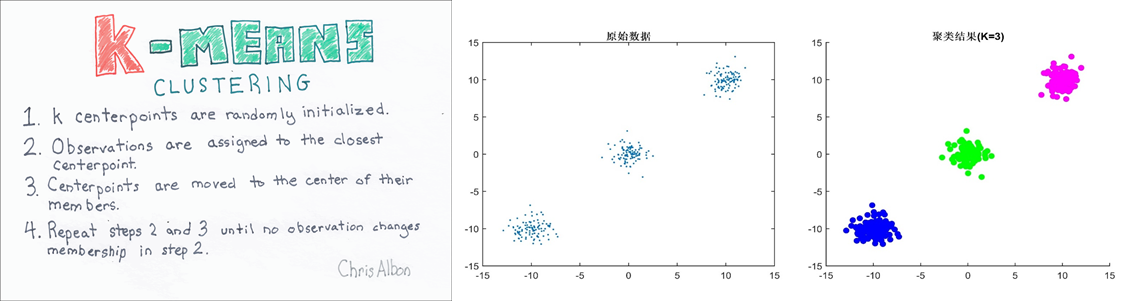KMeans聚类的步骤

随机产生K个点作为各个中心点；
根据数据集中各个点与各个中心点的距离，将数据集归类于各个中心点；
在各个簇内，求出新的中心点（各个点x,y值的平均值）；
循环执行步骤2和3；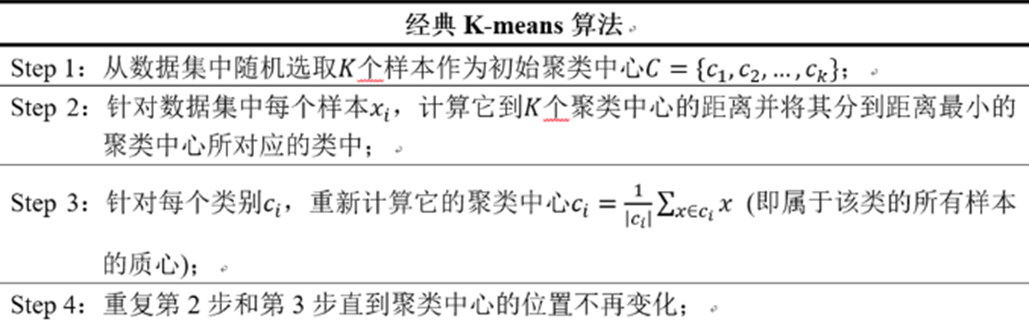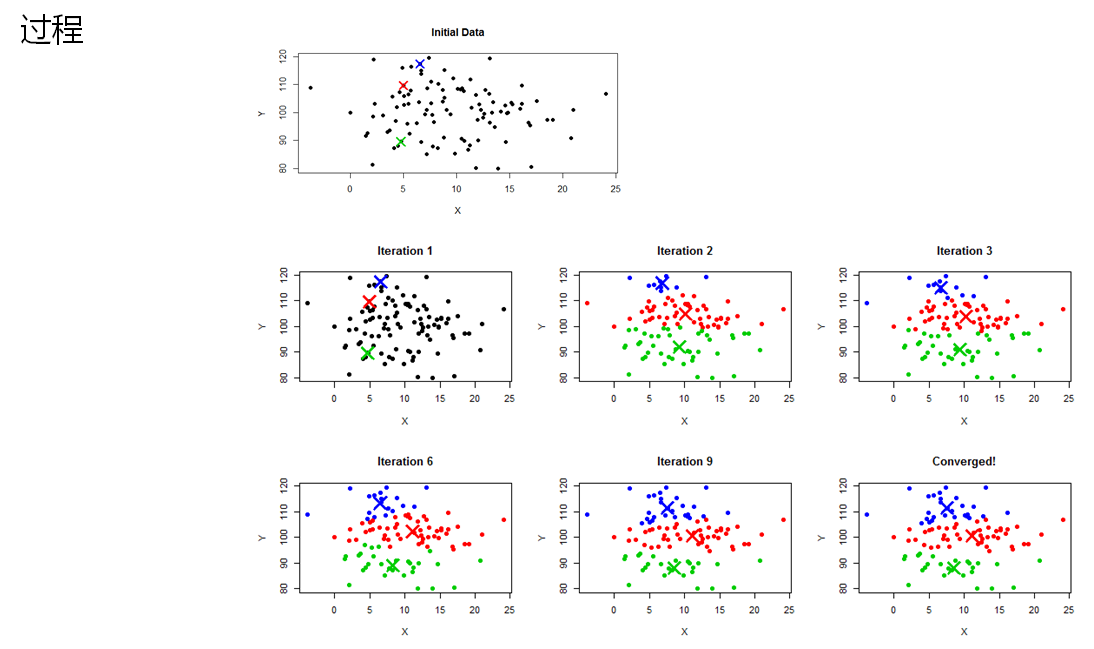Kmeans聚类常见问题及改进

聚类个数是需要人为指定的超参数；
模型初始数据点随机抽取，影响模型稳定性；
每次迭代需要所有数据点依次计算，算法效率低下；
无法对非凸数据集进行可靠聚类；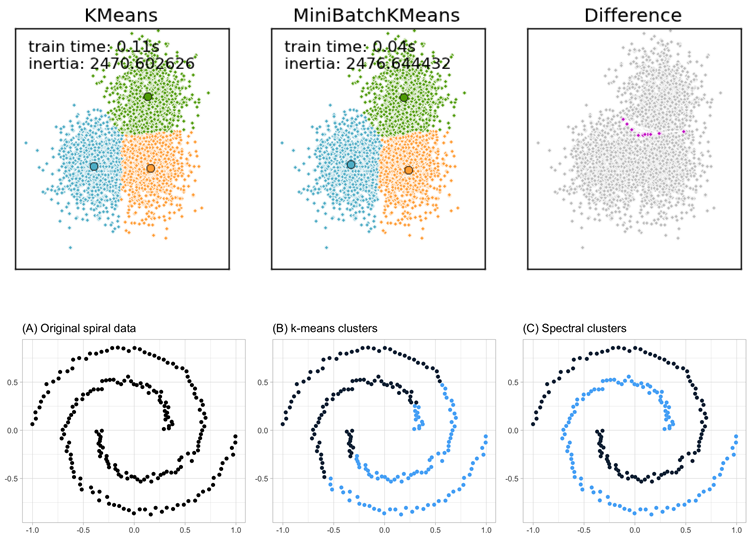Kmeans聚类的变形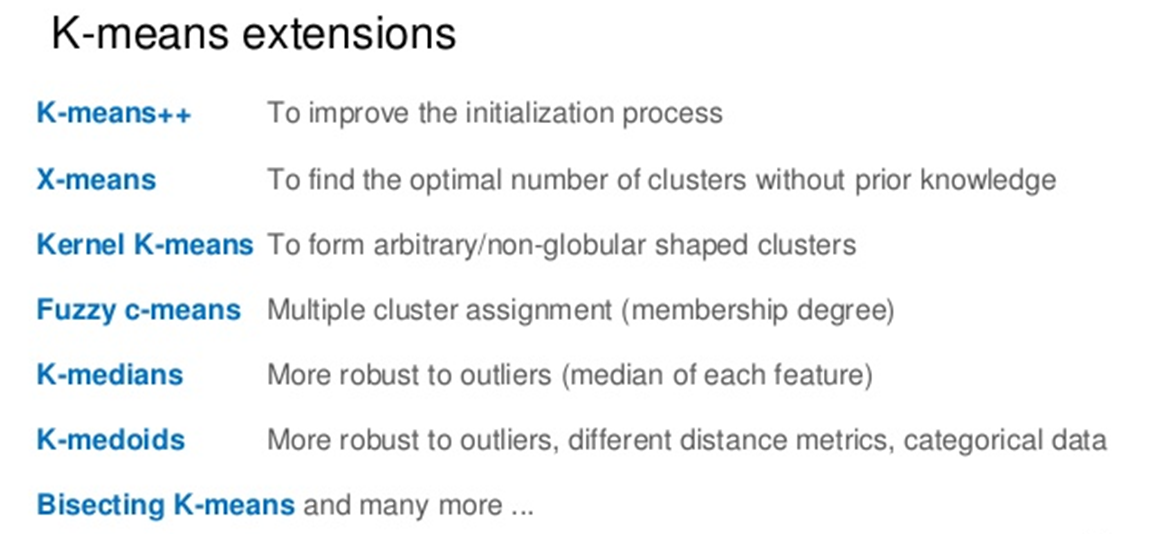Kmeans聚类的优缺点

优点：
算法简单，容易实现 ；
算法速度很快；
对处理大数据集，该算法是相对可伸缩的和高效率的，因为它的复杂度大约是O(nkt)，其中n是所有对象的数目，k是簇的数目,t是迭代的次数。通常k<<n。这个算法通常局部收敛。
算法尝试找出使平方误差函数值最小的k个划分。当簇是密集的、球状或团状的，且簇与簇之间区别明显时，聚类效果较好。

缺点：
对数据类型要求较高，适合数值型数据；
可能收敛到局部最小值，在大规模数据上收敛较慢
分组的数目k是一个输入参数，不合适的k可能返回较差的结果。
对初值的簇心值敏感，对于不同的初始值，可能会导致不同的聚类结果；
不适合于发现非凸面形状的簇，或者大小差别很大的簇。
对于”噪声”和孤立点数据敏感，少量的该类数据能够对平均值产生极大影响。

展开全文深度学习 机器学习 数据挖掘 神经网络
• 鸢尾花的聚类采用的是Kmeans聚类，主要考虑如何将各列特征表示并排列组合，选择2列特征向量时可采用2个for循环，来对所有可能的组合进行遍历，选择3列特征分析时，由于情况较少则可以直接输入列。然后将特征列输入...
• 这是 kmeans 聚类算法的超级快速实现。 代码完全矢量化并且非常简洁。 它比 Matlab 内置的 kmeans 函数快得多。 还包括 kmeans++ 播种算法 (kseeds.m) 以实现良好的初始化。 所以，这个包不仅是为了炫酷，它确实是...matlab
• kmeans聚类的案例，包含测试的模拟数据xml文件，kmeans聚类分析代码
• kmeans 聚类问题实例，用kmeans聚类算法将数据分成三类，实现三分类问题，并将分类结果进行储存机器学习
• Kmeans是我接触的第一个机器学习算法，原理简单，却很实用，只要一想到聚类，基本上没有Kmeans解决不了的问题（此处略有夸张~~），本篇整理了Kmeans聚类原理，评判标准以及Sklearn实现过程 一、Kmeans聚类原理 用...
数据分析目录（文末有超级彩蛋！）：
一、Excel系列——四大常用函数及十大高级图表
二、SQL系列——性能优化/多表关联/窗口分析函数等
三、统计学系列——概率论/置信区间/相关/抽样等
四、Pandas系列——数据读取/清洗/规整/分析实战等
五、Python做图系列——箱型图/散点图/回归图/热力图等
六、业务积累系列——流水预测/精细化运营/排序算法等
七、Kmeans系列——原理/评价指标/RFM实战等
八、决策树系列——算法原理/调参/python实现/项目实战
九、贝叶斯系列——算法原理/3种模型/文本分类实战
十、神经网络系列——BP算法原理/最小二乘法/项目实战
Kmeans是我接触的第一个机器学习算法，原理简单，却很实用，只要一想到聚类，基本上没有Kmeans解决不了的问题（此处略有夸张~~），本篇整理了Kmeans聚类原理，评判标准以及Sklearn实现过程（文末有大礼赠送）
一、Kmeans聚类原理
用大白话来说，Kmeans分为三步：
1、待分类的样本向量化，投射到坐标轴上，先定分几个类（假设3类），随机找3个点做为初始聚类中心，分别计算每个点到3个中心的距离，哪个最近，这个点就属于哪个类了；
2、据第一步分好的类对其内部点求均值，重新做为聚类中心，再计算一遍所有点到这几个中心的距离，重新聚类，这时肯定会有一些点叛逃，没关系，就让他走！ 忠诚的还是会留下来的！
3、就这么一直迭代，直到再也没有点移动或者达到设定的标准了，就可以结束啦！

回想一下我们学过的二维笛卡尔坐标系，如果2个点的横纵坐标差距很小，从空间上看两个点就会挨得特别近，这个其实就是聚类的核心思想，衡量2个点之间差距的就叫做欧氏距离：( (x1-x2)^ 2+(y1-y2) ^ 2 ) ^1/2，扩大到多维，就是各维度坐标相减后求平方和再开方
这里有一个疑问，为什么聚类和分类都是以欧氏距离或者其它距离，而在推荐系统中余弦相似度却用的比较多？
经过深思熟虑之后，我认为主要还是业务需求不同造成的：
聚类是想将用户按特定标准分成几类，然后针对不同的类型采用不同的运营方式，这叫做精细化运营，因此每个指标都很接近的，那必须是一类人了，比如每个月购买金额在10万以上，登录天数在20天以上，这很明显就是很有钱的忠诚用户，这个群体就是必须要小心翼翼地呵护的。而推荐系统其实不是为了找到各方面都很接近的人，而是找到同样喜欢一些东西的人，那喜欢怎么衡量，余弦相似度就是绝佳的评判标准，它只看夹角，不看距离，不管一个月花10万，还是一个月花1千，只要两人买的最多的都是同一件商品，那么就代表两人对这个商品的喜欢程度就是一样的。
二、Kmeans评判标准
Kmeans聚类前要考虑几个问题：
1、数据是否有聚类的趋势
如果是纯随机，那么不管怎么调参，聚类的效果均是不好的，聚类趋势怎么判定，用霍普金斯统计量，取任意N个点与最近向量的距离和，再取N个点与最近向量的距离和，前者除了两者的和，如果纯随机则在0.5附近，有聚类趋势的话会趋近于1或0
2、如何确定分几类？
一般有2种方法，1是经验法，分类数=样本数/2再开根号，当然这也没啥理论依据；另一种更科学的方法叫肘方法，先分1类，2类，3类，N类，分别计算不同分类下所有点到各自聚类中心的距离，可以想到肯定是分1类距离最大，分到N类距离为0（每个点都是一个类），当从1类变成2类，距离会迅速减小，2类变成3类，3类变成4类，直到分到某个数时，发现其减少的量会变得很缓，达到一个拐点，那这个拐点就是最佳的分类数，如果画图就像一个手肘，于是美其名曰：轴方法
3、如何评判分类质量？
不像其它监督学习可以用测试集直接进行质量评判，聚类没有样本输出，但可以根据簇内稠密度和簇外分散度来衡量，一般有2种，而这2种Sklearn中都有。
一个是轮廓系数，向量与簇内部各点距离求均值，衡量簇内部的紧凑程度，再与簇外部所有点的距离求均值，衡量簇外部的分散程度，后者减掉前者，再除了两者的最大值，结果在[-1,1]之间，如果趋近于1，那是分得相当好了，如果是负数，那啥也别说了，直接重来吧~~在Sklearn中是用silhouette_score()计算所有点的平均轮廓系数，而silhouette_samples()返回每个点的轮廓系数
另一个是Calinski-Harabaz（CH），用的是簇间的协方差矩阵与簇内的协方差矩阵相除，如果前者越大，后者越小，那么分值越大，分类越好，在sklearn中et是是用metrics.calinski_harabaz_score.
三、Sklearn实现
1、主要参数介绍

n_clusters: K值，这个值一般需要结合第3点的评判标准，找到最佳的K
2）max_iter： 最大的迭代次数，一般如果是凸数据集的话可以不管这个值，如果数据集不是凸的，可能很难收敛，此时可以指定最大的迭代次数让算法可以及时退出循环。
3）n_init：用不同的初始化质心运行算法的次数。由于K-Means是结果受初始值影响的局部最优的迭代算法，因此需要多跑几次以选择一个较好的聚类效果，默认是10，一般不需要改。如果你的k值较大，则可以适当增大这个值。
4）init： 初始值选择的方式，一般默认’k-means++’。

2、使用数据生成器生成聚类数据，采用CH分数和散点图评判聚类结果
from sklearn.cluster import KMeans
from sklearn.datasets import make_blobs
from sklearn import metrics
import matplotlib.pyplot as plt
from sklearn import datasets

x,y = make_blobs(n_samples=1000,n_features=2,centers=4,cluster_std=[0.2,0.3,0.5,0.4],shuffle=True,random_state=9)
print(x[:5])
score = []

fig = plt.figure(figsize=(20,20))
ax1 = fig.add_subplot(221)
plt.scatter(x[:,0],x[:,1],c=y)
plt.title('原始（设定分4类）')

ax2 = fig.add_subplot(222)
clf = KMeans(n_clusters=3,max_iter=1000)
pred = clf.fit_predict(x)
score.append(metrics.calinski_harabaz_score(x,pred))
plt.scatter(x[:,0],x[:,1],c=pred)
plt.title('Kmeans分3类')

ax3 = fig.add_subplot(223)
clf = KMeans(n_clusters=4,max_iter=1000)
pred = clf.fit_predict(x)
score.append(metrics.calinski_harabaz_score(x,pred))
plt.scatter(x[:,0],x[:,1],c=pred)
plt.title('Kmeans分4类')

ax4 = fig.add_subplot(224)
clf = KMeans(n_clusters=6,max_iter=1000)
pred = clf.fit_predict(x)
score.append(metrics.calinski_harabaz_score(x,pred))
plt.scatter(x[:,0],x[:,1],c=pred)
plt.title('Kmeans分6类')
plt.rcParams['font.sans-serif'] = ['SimHei']
plt.rcParams['font.serif'] = ['SimHei']  # 设置正常显示中文
plt.show()
print(score)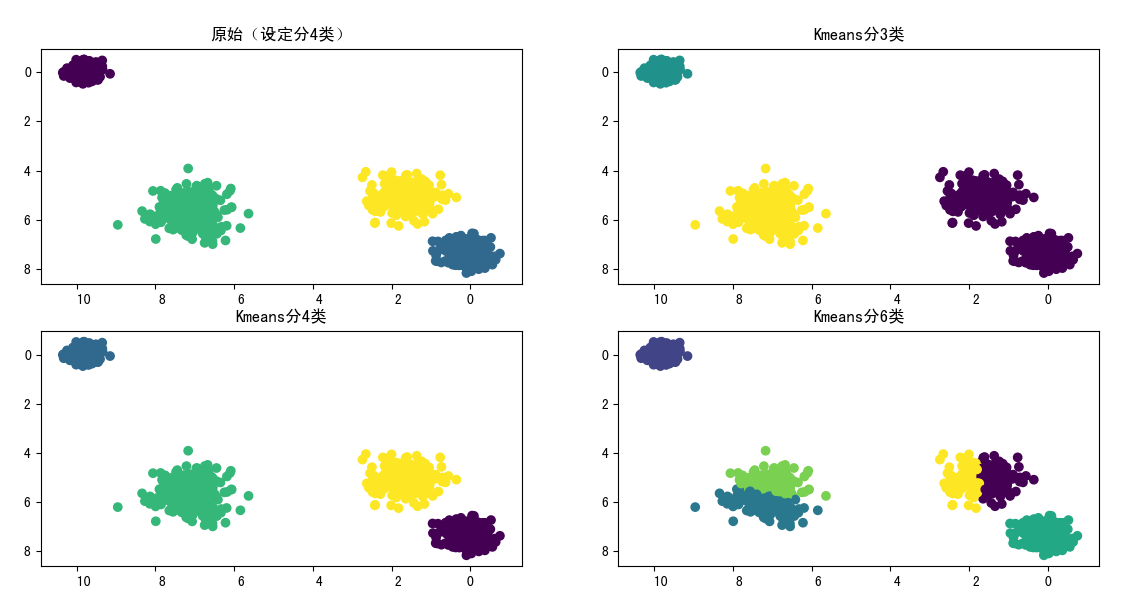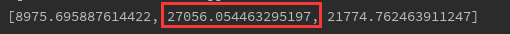从CH分数看，分4类是最高的，这与预期设定的4类结果是一致的，从散点图上也能看出，分4类也是最好的。当特征超过2维后，我们肉眼已经无法直观判断时，CH分数可以做为很实用的替代方法

本人互联网数据分析师，目前已出Excel，SQL，Pandas，Matplotlib，Seaborn，机器学习，统计学，个性推荐，关联算法，工作总结系列。

微信搜索 " 数据小斑马" 公众号，回复“数据分析"就可以免费领取数据分析升级打怪 15本必备教材喔展开全文轮廓系数
• KMeans聚类实验，内含相关代码和程序已打包，还有实验报告word,够您使用。KMeans聚类实验，内含相关代码和程序已打包，还有实验报告word,够您使用。KMeans聚类实验，内含相关代码和程序已打包，还有实验报告word,够...
• kmeans聚类算法，目前只支持一位数组。亲测可用。
• KMEANS聚类工具，高效，可以自动确定聚类个数。聚类准确度高
• 期末作业，Kmeans聚类分类动漫数据，进行简单的数据归类，解压后用IDEA可直接运行，很简陋的程序，内行看笑话，勿喷
• kmeans.py，python算法之Kmeans聚类分析
• 主要介绍了Python实现的KMeans聚类算法,结合实例形式较为详细的分析了KMeans聚类算法概念、原理、定义及使用相关操作技巧,需要的朋友可以参考下
• java实现的kmeans聚类算法, 对某张表的某个字段进行kmeans聚类算法,并写到新创建的表中
• 主要为大家详细介绍了Python KMeans聚类问题，具有一定的参考价值，感兴趣的小伙伴们可以参考一下
• 主要为大家详细介绍了Python实现Kmeans聚类算法，具有一定的参考价值，感兴趣的小伙伴们可以参考一下
• Python实实现现Kmeans聚聚类类算算法法 这篇文章主要为大家详细介绍了Python实现Kmeans聚类算法文中示例代码介绍的非常详细具有一定的参考价 值感兴趣的小伙 们可以参考一下 本本节内内容容 本节内容是根据上学期所...
•python...